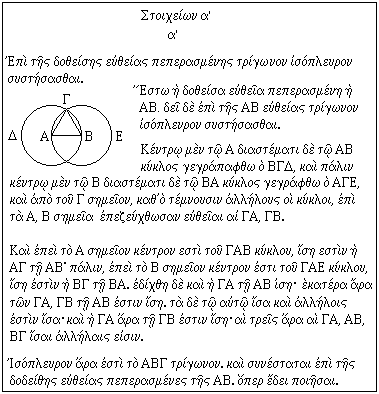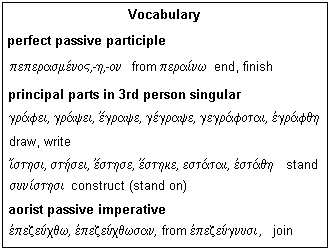## Lesson θ': The Euclidean Proposition

We see how a proof is laid out, and study Book I, Proposition 1 of the Elements as our example.

The Elements consists of definitions, postulates, axioms, and propositions or demonstrations. The latter are of two types, problems and theorems, and are set out in a standard format, which is the same for both. The parts of a demonstration are as follows:

1. Statement of the Theorem or Problem
2. The Figure
3. Application to the Figure
4. Constructions
5. Proof
6. Final statement of what has been proved

At the end of every proposition there is a short conventional phrase saying that what was proposed to be done has now been done. In Latin, a theorem ends with quod erat demonstrandum, "which was to be shown", Q.E.D., and a problem ends with quod erat faciendum, "which was to be done", Q.E.F. The corresponding phrases in Greek are shown in the box below.The first word is the intensified relative pronoun, neuter singular accusative. By itself, o/( means "that", but adding the intensifier -περ makes it say "precisely that" or something similar. The case is accusative because the pronoun is the object of the infinitive at the end of the phrase.

The second word comes from the verb "to bind" and is used in the third person singular to imply "to be necessary". The form used is the imperfect tense, like the Latin erat. The imperfect describes an action continuing or repeated in the past. It is the typical past tense of "to be". The state of being necessary is certainly continuous, and began at some indefinite time, so the imperfect is the only tense that would do here. To make the imperfect, we augment (and the accent springs ahead) and add the proper personal endings. Here, the third person singular ending is ε (instead of ει as is used in the present tense). Finally, contraction gives us the word.

The third word corresponds to demonstrandum. In Latin, this means precisely "to be demonstrated", and agrees with quod, but in Greek we use an infinitive instead, introduced by the verb implying the necessity. The verb is an -μι verb whose six principal parts are given. Only the present tense is conjugated with different endings than we are used to, and you should be able to form the future and aorist active without trouble. The fourth principal part is the perfect active, the fifth the perfect middle, and the sixth the aorist passive. The stem of the verb ends in -κ which combines with a following σ to give ξ. You should not be stumped by this sort of change any longer. Where it appears, the -νυ- is inserted for euphony. There is, in fact, a present tense using the -ω endings we are familiar with that was an alternative to the -μι forms.

The infinitive is aorist, not future. A future active infinitive would end in -σειν, not -σαι, as this one does. Although the proving is in the future relative to the necessity, in Greek this does not call for the future. The action of proof is a single action, which will be carried out once and then will be finished. This demands the aorist, whenever the deed happens. The same thing happens for the Q.E.F., but you already know how to conjugate poie/w, taking care to contract the final ε of the stem with the vowels of the endings. The method of building the aorist active infinitive is shown in the box. The circumflex is characteristic of the aorist active infinitive.

A casual look at QED might conclude that the pronoun is nominative, the verb is present, and the infinitive is future. Analysis reveals all of these assumptions are incorrect, and that the truth is much more interesting.Let us now study the very first proposition in the Elements, Book 1, Proposition 1, which is shown in the box. This is a problem, and is necessary for showing that the postulates imply that a line of given length can be moved to any point, which is Proposition 2, and then that a distance equal to any given line can be cut off on any other given line, which is Proposition 3. This proves rigorously that it is permissible to use dividers to transfer a distance. This mechanical procedure is subject to error, but Euclid proves that, in principle, it can be done exactly.

The first paragraph is the statement of the problem. A literal translation is: onto the given straight terminated (perfect) a triangle equilateral to be constructed (aorist). In better English, to construct an equilateral triangle on a given finite straight line. The word for terminated is a perfect passive participle (compare with pe/raj, end). The reduplication is a giveaway of the perfect tense. Perfect is used here because once the line is terminated, the effect persists ever after. The infinitive is an aorist passive infinitive of the verb suni/sthmi, of which the sign is sqai, as sai is of the active, as we saw above.

We see the figure, and the text says: Let there be the given straight terminated (perfect) the AB. It is necessary indeed upon the AB straight a triangle equilateral to be constructed (aorist). That is, Let AB be the given finite line. It is required to construct an equilateral triangle upon it. The same participles and infinitives are used.

Now we proceed to the construction. The text is: At the center A to interval AB let a circle be drawn (perfect) the BΓΔ, and likewise at center B to interval BA let a circle be drawn (perfect) the AΓE, and from the Γ point, under which cut each other the circles, to the A, B points let be joined (aorist) straights the ΓA, ΓB. That is, Draw a circle with center A and radius AB, and another with center B and radius BA. Join the point of intersection of the circles Γ and points A, B.

In the Greek text, no reference is made to previous propositions, but in modern translations it is the custom to note the justification for each step. For example, Postulate 3 says that a circle can be drawn from any center with any radius, and Postulate 1 that a straight line can be drawn joining any two points. This the justification for the construction we have just made. Of course Euclid would know this, and any student who made an unjustified move would be brought up quickly.The construction done, we can proceed to the proof. The text says: and since the A point center is of the ΓΔB circle, equal is the AΓ to the AB; likewise, since the B point center is of the ΓAE circle, equal is the BΓ to the BA. it was shown (aorist) also the ΓA to the AB equal; each therefore of the ΓA, ΓB to the AB is equal. The to the same equal also to each other is equal; and the ΓA therefore to the ΓB is equal; the three therefore the ΓA, AB, BΓ equal to each other are. In English, this is: Since A is the center of circle GDB, AG = AB; likewise, since B is the center of circle GAE, BG = BA. But GA was shown equal to AB, so each of GA, GB are equal to AB. Things equal to the same things are equal to each other, so GA = GB. Therefore, the three lines GA, AB, BG are equal to each other. Note that a singular verb is used with plural neuters, but plural masculine and feminine always call for a plural verb. This is a peculiarity of Greek.

It is only necessary now to draw the conclusions. The text is: Equilateral therefore is the ABΓ triangle. And it has been constructed (perfect) upon the given straight terminated (perfect) the AB. Which was to be done. In English, Triangle ABG is equilateral, and constructed upon the given line AB, which was to be done.

The tenses of the participles and imperatives have been given; note that they are either aorist or perfect, and that the distinction is not one of time, but of the kind of action involved. Once a line is terminated it is terminated forever (perfect), but when something is proved, the action is complete (aorist). It is good that we do not have to decide which to use, but merely to interpret what is in front of us. The vocabulary box above shows the perfect passive participle for "finite". This participle can be recognized by the reduplication of the stem, and the accent on the penult, so it can be distinguished from the present passive participle with the accent on the antepenult. The box also gives the principal parts of the verbs for draw and stand, with the endings for the third person singular instead of the usual first person. We see these endings more, and should be better acquainted with them. Note that the sixth principal part, the aorist passive, is the one we frequently use both for participles and imperatives. An example of the aorist imperative is given.

### Exercises

1. If you already know some Greek, it will be easy to read the proposition once you have grasped the peculiarities of the language used, with all its third person imperatives and perfect participles. If you are learning, the text will repay word-for-word study, and any time you spend will not be wasted. This demonstration looks very much like all of Euclid's demonstrations, and most of the words are very commonly used indeed. Take each word, and find out why it has the ending it does, and how it is used. Write out the proposition yourself, and read it aloud. The literal translation into English is, of course rather ridiculous, but the reason is that English does not have the cases and tenses. The language is really quite elegant and precise, and you will come to appreciate it.

2. Translate the proposition into good English, and justify all statements, which here can be only by reference to the definitions, postulates and axioms.